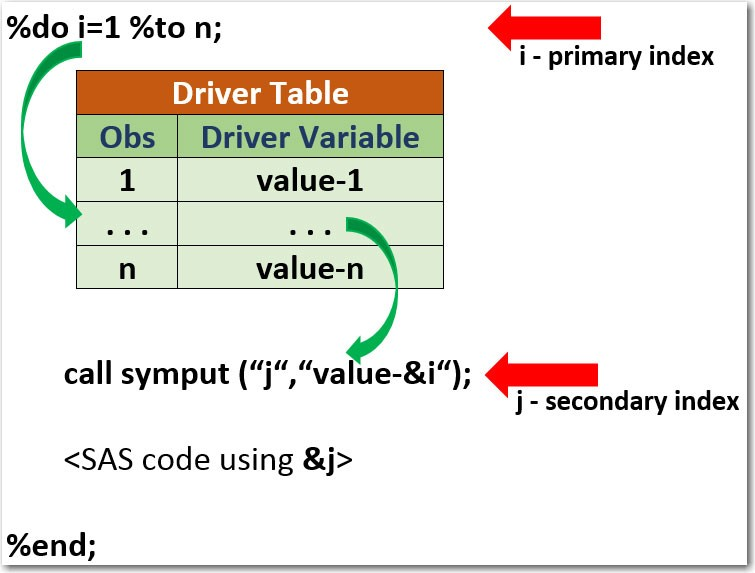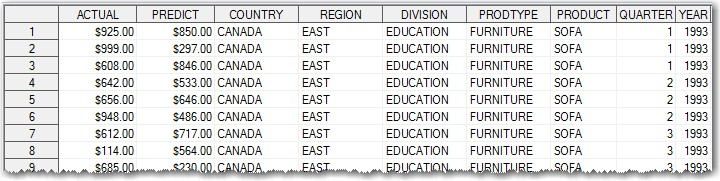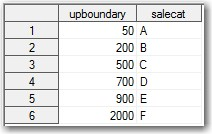In my previous post, Introducing data-driven loops, I suggested a way of implementing programming loops with a list of index variables pulled from an external data table. These ordinary programming loops iterate during code execution while processing some data elements of an input data table.

SAS macro loops, on the other hand, are completely different creatures as they do not iterate during execution time, but rather during code compilation time. That makes SAS macro loops a powerful code generator tool allowing to produce multiple variations of SAS code snippets with actually writing them just once.

Syntactically speaking, while SAS programming loops or do-loops always reside within SAS data step, SAS macro loops or %do-loops are located within SAS macros. They can be either within a data step (or proc step) generating multiple data/proc step statements, or outside data/proc step generating multiple data/proc steps or global statements.

### Implementing SAS macro loops

To make macro loop driven by data we can use two index macro variables: the first one (primary index) iterates from 1 to n incrementing by 1 effectively going through the observations of a driver table, the other macro variable (secondary index) gets its values from the driver variable and is being a true data-driven index for our macro loop.  The following figure illustrates this concept.### SAS macro loops containing data or proc steps

For example, we have data table sashelp.prdsale that looks like this:Suppose, we need to produce in separate HTML files for each country - charts showing actual product sales by years.

Here is how this can be done the data-driven way without any hard-coding:

```/* output files location */
filename odsout "C:PROJECTS_BLOG_SASdata-driven-macro-loopshtml";

/* get unique countries */
proc sort data=sashelp.prdsale(keep=COUNTRY) out=work.countries nodupkey;
by COUNTRY;
run;

%macro loop;

%local num i cntry;

/* get number of countries */
%let dsid = %sysfunc(open(work.countries));
%let num  = %sysfunc(attrn(&amp;dsid,nlobs));
%let rc   = %sysfunc(close(&amp;dsid));

%do i=1 %to #

data _null_;
p = &amp;i;
set work.countries point=p;
call symputx('cntry',COUNTRY);
stop;
run;

ods html path=odsout file="report_&amp;cntry..html"  style=styles.seaside;
goptions reset=all device=actximg colors=() htext=9pt hsize=5in vsize=3in;
title1 "Product sales by year for &amp;cntry";
axis1 minor=none label=('Actual Sales');

proc gchart data=sashelp.prdsale(where=(COUNTRY eq "&amp;cntry"));
vbar YEAR /
sumvar = ACTUAL
width = 10
outside = sum
raxis = axis1
cframe = white nozero discrete
;
format ACTUAL dollar12.0;
run;
quit;

ods html close;

%end;

%mend loop;
%loop;
```

The highlights of this code are:

1. Using proc sort with nodupkey option we create a table work.countries of unique COUNTRY values. This can be done using proc sql as well.
2. We determine the number of unique COUNTRY values, &num.
3. Within macro called %loop, we use primary index – macro variable &i – to iterate from 1 to &num with increment 1.
4. We use data _null_ step within that loop to sequentially read values of COUNTRY using direct access to observations of work.countries table by means of point= option. For each iteration &i of %do-loop, we create a secondary index – macro variable &cntry, which is used as a true index for our loop.
5. During the code compilation, SAS macro processor loops through the %do-loop &i times repeatedly generating SAS code within it, each time with a new value &cntry, thus accomplishing our task.

This implementation of the macro %do-loop works perfectly fine, except in the situations when we need to use it within a data/proc step. The problem is the data _null_ statement that converts primary index &i to the secondary index &cntry, since we can’t use a data step within another data step.

### SAS macro loop within data or proc step

Let’s solve the following coding problem. Suppose we have to create SALE_CATEGORY variable on our sashelp.prdsale table, something that you would routinely code like this:

```data work.prdsale;
set sashelp.prdsale;
if ACTUAL &lt; 50 then SALE_CATEGORY = 'A'; else
if ACTUAL &lt; 200 then SALE_CATEGORY = 'B'; else
if ACTUAL &lt; 500 then SALE_CATEGORY = 'C'; else
if ACTUAL &lt; 700 then SALE_CATEGORY = 'D'; else
if ACTUAL &lt; 900 then SALE_CATEGORY = 'E'; else
if ACTUAL &lt; 2000 then SALE_CATEGORY = 'F';
run;
```

What is wrong with this code? Nothing. Except when category definition changes you would have to find every place in your code where to apply that change. Besides, if a number of categories is large, the code becomes large too.

Let’s implement this the data-driven way, without any hard-coded values. Notice, that in the code above we have multiple if-then-else statements of a certain pattern that are repeated multiple times and thus they can be generated via %do-loop.

Let’s create the following driver table that contains boundary and sale category definitions that match the above hard-coded data step:The data-driven macro loop can be implemented using the following code:

```%macro mloop;

/* get observations number - num, variable numbers - vnum1, vnum2, */
/* variable type - vtype2, getfunc = getvarC or getvarN            */
%let dsid = %sysfunc(open(work.salecategory));
%let num  = %sysfunc(attrn(&amp;dsid,nlobs));
%let vnum1 = %sysfunc(varnum(&amp;dsid,upboundary));
%let vnum2 = %sysfunc(varnum(&amp;dsid,salecat));
%let vtype2 = %sysfunc(vartype(&amp;dsid,&amp;vnum2));
%let getfunc = getvar&amp;vtype2;

data work.prdsale;
set sashelp.prdsale;

%do i=1 %to #

/* get upboundaty and salecat values from driver table work.salecategory */
/* and assign them to upper and categ macro variables */
%let rc = %sysfunc(fetchobs(&amp;dsid,&amp;i));
%let upper = %sysfunc(getvarn(&amp;dsid,&amp;vnum1));
%let categ = %sysfunc(&amp;getfunc(&amp;dsid,&amp;vnum2));
%if &amp;vtype2 eq C %then %let categ = "&amp;categ";

/* generate if ... then ...; else statements */
if ACTUAL &lt; &amp;upper then SALE_CATEGORY = &amp;categ;
%if (&amp;i ne &amp;num) %then %str(else);

%end;
%let rc = %sysfunc(close(&amp;dsid));

run;

%mend mloop;
%mloop;
```

With a little overhead of several %sysfunc() functions and SAS Component Language (SCL) functions we effectively generate a set of if-then-else statements based on the values in the driver table. Notably, even if the number of categories increases to hundreds the code does not have to be changed a bit.

Of course, this approach can be used for any SAS code generating efforts where there is a repetition of SAS code pattern.

### Bonus

As a bonus to those who was patient enough to bear with me to the end, here is a macro equivalent of the data _null_ statement used in the first section - SAS macro loops containing data or proc step:

Data step:

```data _null_;
p = &amp;i;
set work.countries point=p;
call symputx('cntry',COUNTRY);
stop;
run;
```

Macro equivalent:
```%let dsid = %sysfunc(open(work.countries));
%let vnum = %sysfunc(varnum(&amp;dsid,COUNTRY));
%let rc = %sysfunc(fetchobs(&amp;dsid,&amp;i));
%let cntry = %sysfunc(getvarc(&amp;dsid,&amp;vnum));
%let rc = %sysfunc(close(&amp;dsid));
```

### Thoughts